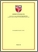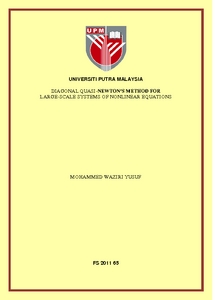# Diagonal quasi-newton's method for large-scale systems of nonlinear equations

## Citation

Yusuf, Mohammed Waziri (2011) Diagonal quasi-newton's method for large-scale systems of nonlinear equations. PhD thesis, Universiti Putra Malaysia.

## Abstract

The famous and well known method for solving systems of nonlinear equations is the Newton’s method. This method is simple to implement and converges rapidly. Nevertheless, an iteration of this method turns out to be computational expensive,this is due to the fact that the method requires the computation and storage of the Jacobian matrix, the floating point operations is O(n)3 and as well as the solution of an n linear systems in each iteration. In addition the convergence may even be lost when the Jacobian is singular at a solution. These drawbacks/disadvantages is more noticeable when handling large-scale systems of nonlinear equations. This leads to the idea of this thesis. This thesis focuses on some variants of Newton’s method for solving systems of nonlinear equation, especially large-scale systems. Several diagonal Newton’s methods for solving systems of nonlinear equations have been derived. Unlike the classi cal Newton’s method, the proposed schemes require neither the computation and storage of the Jacobian matrix, nor the solution of a system of linear equations in each iteration. Thus, the floating point operations have been reduced to O(n). This is made possible by approximating the Newton step via diagonal updating. The basic idea behind this novel approach is to approximate the Jacobian or its inverse to a nonsingular diagonal matrix which can be updated in each iteration. The anticipation of the methods proposed in this thesis when applied to large-scale systems of nonlinear equation has been in the reduction of computational costs, storage requirements, floating points operations and processing time. Extensive computational experiments are performed on standard problems to examine the impact of the proposed methods compared to other variants of Newton’s method for solving systems of nonlinear equations. The results suggest that the proposed methods outperform the classical Newton’s method and some of its variants in terms of matrix storage requirements, computational cost and processing time in seconds. In addition, the local convergence of the proposed methods have been proven. We further apply the diagonal updating schemes proposed in this thesis to handle some well known real-life problems and compare their numerical performance with some Newton-like methods. Finally, we discuss some possible extension of our work.Preview
PDF
FS 2011 65R.pdfView Item